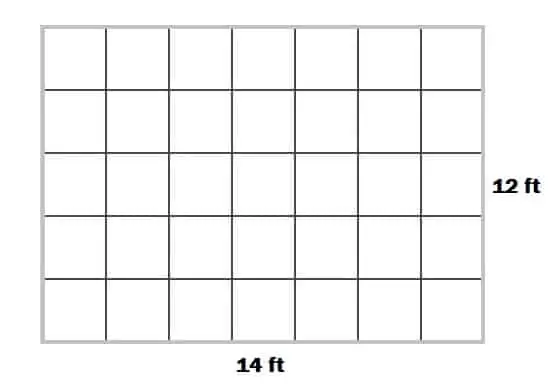# How To Calculate Number Of Tiles In A Room

Contents

## How To Calculate Number Of Tiles In A Room:

Calculation of tiles needed for floors and walls is so simple and easy. in this post, I will discuss how to calculate no. of tiles required in a room. So let’s start.

## Step 1- Calculate Area Of The Floor:let the length of the floor = 14 feet.

Breadth of the floor = 12 feet.

∴ Area of floor = length of floor x breadth of floor = 14 x 12 = 168 sft.

## Step 2 – Calculate Area Of 1 Tile:

There are different tile sizes such as 20cmx20cm, 30cmx30cm, 45×45, 60cmx60cm etc. In this calculattion we will use 60×60 cm tiles.

Length of 1 tile = 60 cm

Breadth of 1 tile = 60 cm.

∴ Area of 1 tile =  60 x 60 = 3600 cm² = 3.6 sft.

## Step 3 – Divide Floor Area By Area Of 1 Tile:

∴ No. of tiles required = Area of floor/ area of 1 tile = 168/3.6 = 46.6 ≈ 47.

∴ 47 tiles are required for the above floor.

Note:

Consider 5% wastages.

Similarly, you can also calculate tiles needed for walls.

### 10 thoughts on “How To Calculate Number Of Tiles In A Room”

1. Alot of thanks to our bloved for ,owning A loving heart giving out what you own.

2. Your engineering journal are highly appreciated. Thanks.

3. So much useful i am working as a finishing engineer please suggest me finishing tips

4. Thank you very much
So simple analysis Need more tips
About block, layout and concrete tips
May God increases you in knowledge Amen

5. I stand to b corrected, I think one step is missing, you can’t just go to d market nd ask for 47 tiles, it’s sold in cartons, I think this should be d last step, make enquiries to know d total numbers of tiles in one carton (60*60cm tile) then divide d total tiles to b used by d numbers of tiles in one carton to get d numbers of cartons. Let’s say we have 10 of 60*60cm tiles in one carton, so 47/10=4.7cartons. thanks.

6. I dont think 3600cm² will be 3.6ft², the calculation might be wrong, we can first convert the ft to cm before proceeding to calculate the area in cm² just like that. How ft makes a cm or how many cm makes a ft.

7. You are wise man ur guidance is simple and understandable congrats and keep it up.

• Thanks, keep supporting.

8. The calculation is wrong
14 ft by 12 ft is approximately 4.2m by 3.6m which is approximately 15.12 sqm.
The area of a 60/60 tile is 0.36sqm.
Therefore 15.12÷0.36 = 42 tiles

In other way
14 ft ÷ 2 = 7 tiles for the length
12 ft÷ 2 = 6 tiles for breadth
7*6 = 42